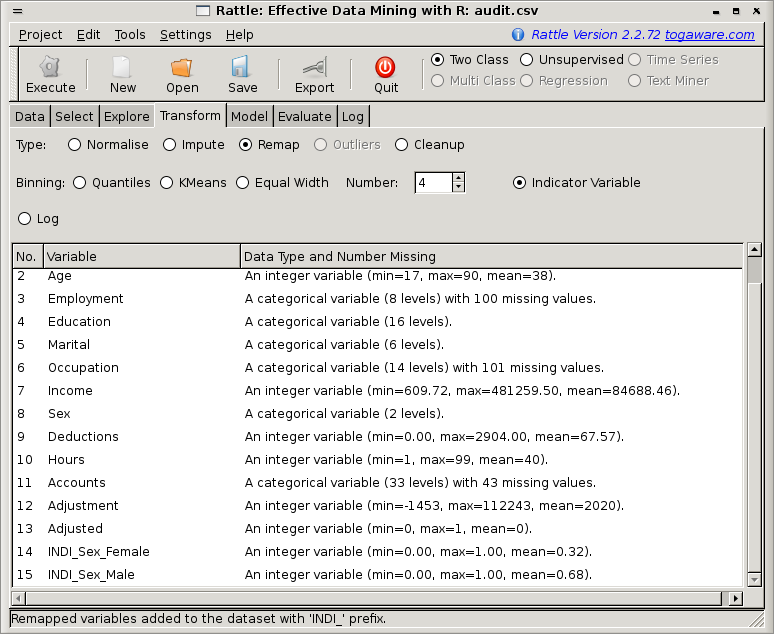DATA MINING
Desktop Survival Guide
by Graham Williams## Indicator Variables

Some model builders do not handle categoric variables. Neural networks and regression are two examples. A simple approach in this case is to turn the categoric variable into some numeric form. If the categoric variable is not an ordered categoric variable, then the usual approach is to turn the single variable into a collection of so called indicator variables. For each value of the categoric variable there will be a new indicator variable which will have the value 1 for any observation that has this categoric value, and 0 otherwise. The result is a collection of numeric variables.

Rattle's Transform tab provides an option to transform one or more categoric variables into a collection of indicator variables. Each is prefixed by `INDI_` and the remainder is made up of the name of the categoric variable (e.g., Gender) and the particular value (e.g., `Female`), to give `INDI_Gender_Female`. Figure 23.9 shows the result of turning the variable Gender into two indicator variables.

There is not always a need to transform a categoric variable. Some model builders, like the regressions in Rattle, will do it for us automatically.